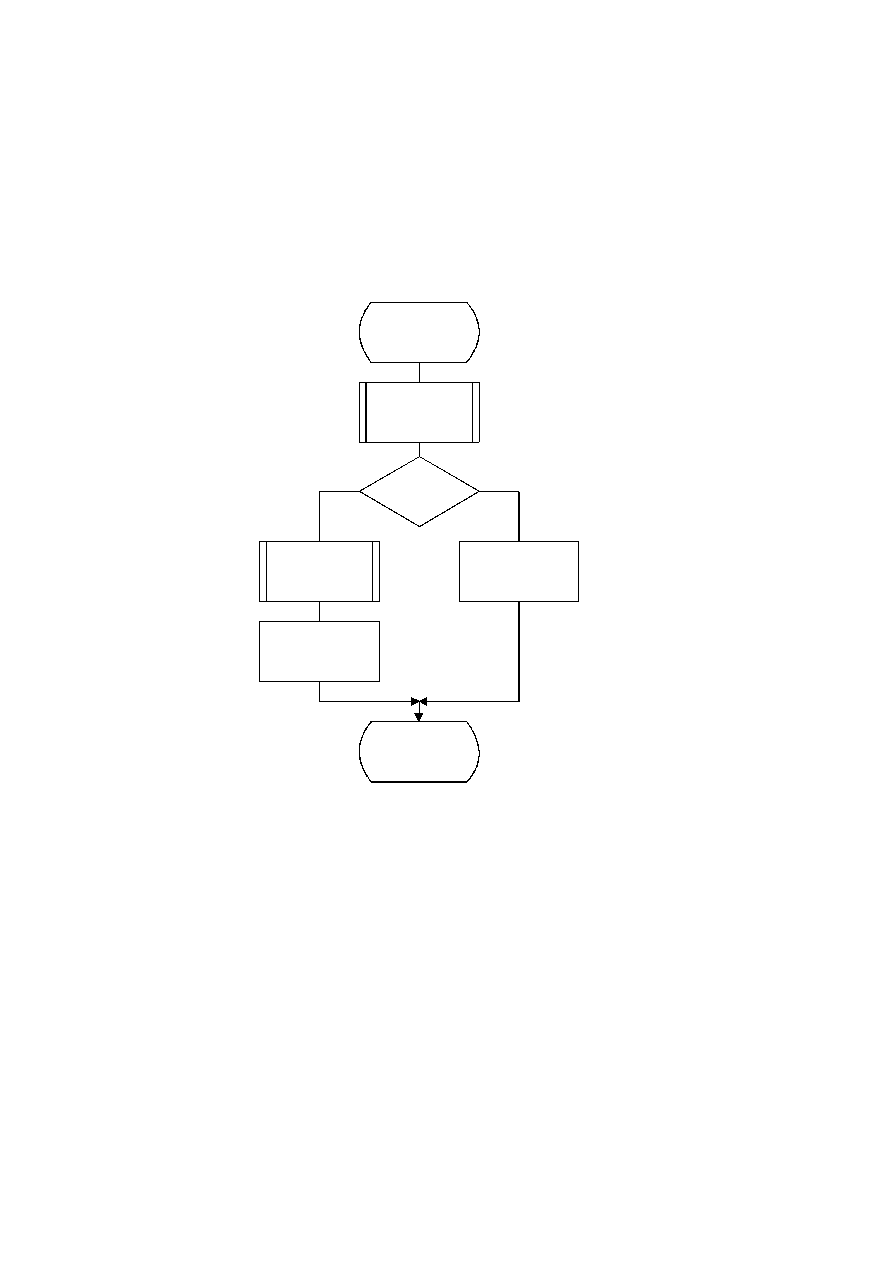ISO/IEC 10918-1 : 1993(E)
The statistical models defined in F.1.4.4 also apply to this decoding process.
The decoder shall be capable of using up to four DC and four AC conditioning tables and associated statistics areas within
one scan.
F.2.4.1
Arithmetic decoding of DC coefficients
The basic structure of the decision sequence for decoding a DC difference value, DIFF, is shown in Figure F.19. The
equivalent structure for the encoder is found in Figure F.4.
TISO1510-93/d089
Decode_DC_DIFF
D = Decode(S0)
D = 0
?
No
Yes
Decode_V(S0)
DIFF = 0
DIFF = V
Done
Figure F.19 ­ Arithmetic decoding of DC difference
Figure F.19 [D89] = 13 cm = 508 %
The context-indices used in the DC decoding procedures are defined in Table F.4 (see F.1.4.4.1.3).
The "Decode" procedure returns the value "D" of the binary decision. If the value is not zero, the sign and magnitude of
the non-zero DIFF must be decoded by the procedure "Decode_V(S0)".
F.2.4.2
Arithmetic Decoding of AC coefficients
The AC coefficients are decoded in the order that they occur in ZZ(1,...,63). The encoder procedure for the coding process
is found in Figure F.5. Figure F.20 illustrates the decoding sequence.
112
CCITT Rec. T.81 (1992 E)